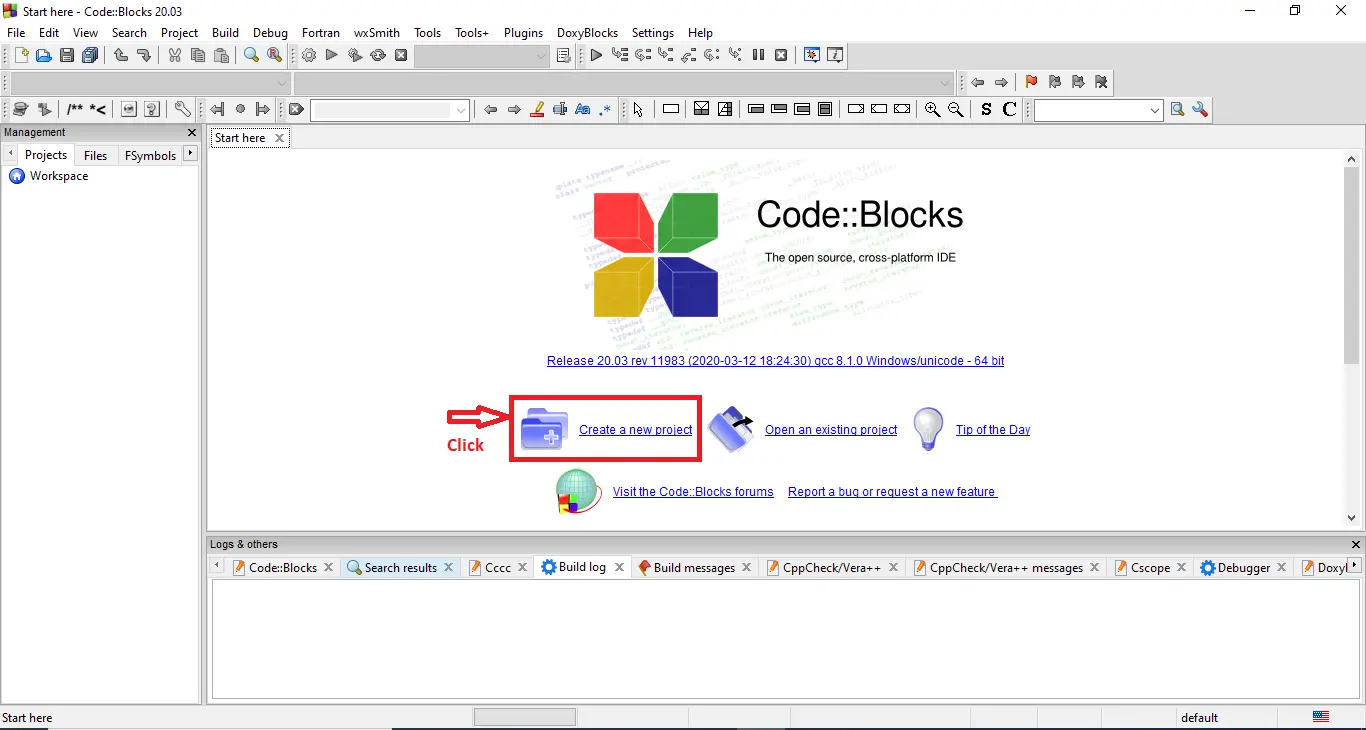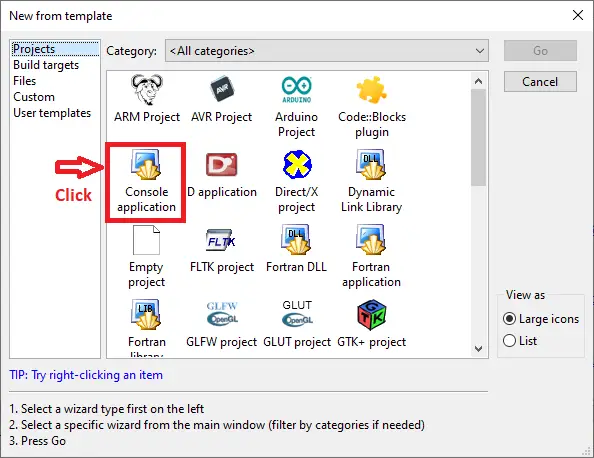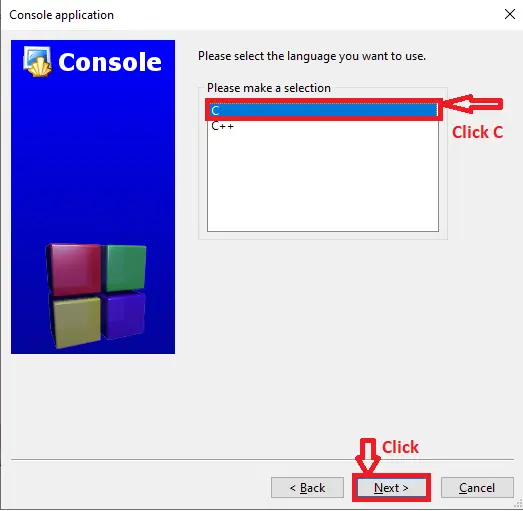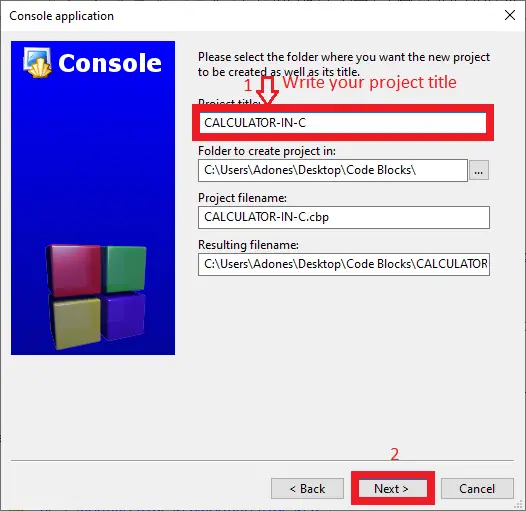# Calculator in C with Source Code

You will learn how to make a simple calculator program in C in this article. This simple calculator is one that can perform addition, subtraction, multiplication, and division, modulus, power and factorial as well as other fundamental mathematical operations.

There are two ways to create a calculator program. The first is by creating a switch case for the program and the second is by creating a function that takes the operator +, -, and – as its operands.

This Calculator C also includes a free Download Source Code. Simply locate the downloadable source code below and click the “Download Now” button. To start creating a C on calculator Language, make sure that you have a Code Blocks or any platform of C installed in your computer.

## Calculator program in C Steps on How to Create a Project

Time needed: 5 minutes.

Here’s the step’s on how to create a Calculator in C with Source Code.

• Step 1: Create a new project.

First open the code blocks IDE and click “create a new project“.• Step 2: Choose console application.

Next, click the “console application” and after that click “next“.• Step 3: Choose C language.

Then , choose “C language” and click “next“.• Step 4: Name your project.

Lastly, name the project you’ve created and click “next” after that click “finish“.• Step 5: The actual code.

Finally, we will now start adding functionality to our C Framework by adding some functional codes.

## This Calculator C with Source Code was build and run under Code::Blocks IDE.

The following is the first screenshot of the sample run:

Now provide any input (from +,-,*,/,?,^,!) in such a way that:
Enter – symbol for Subtraction
For Multiplication Enter * symbol
Enter / symbol for Division
Enter ? symbol for Modulus
Enter ^ symbol for Power
Enter ! symbol for Factorial

• Output for main window

If the user selects * symbol, the application will ask the user to enter any two numbers to accomplish the multiplication. For example, if the user select * symbol and enter 4 as the first number, the second number will be 5. To see the output, press the ENTER key as shown in the screenshot below:

• Output for multiplication window

If the user selects + symbol, the application will ask the user to enter any two numbers to accomplish the multiplication. For example, if the user select + symbol and enter 10 as the first number, the second number will be 12, the third number will be 13 and the fourth number will be 12. To see the output, press the ENTER key as shown in the screenshot below:

If the user selects – symbol, the application will ask the user to enter the number of elements you want to add to accomplish the subtraction. For example, if the user select – symbol and enter 45 as the first number, the second number will be 20. To see the output, press the ENTER key as shown in the screenshot below:

• Output for subtraction window

If the user selects / symbol, the application will ask the user to enter the number of elements you want to add to accomplish the division. For example, if the user select / symbol and enter 39 as the first number, the second number will be 3. To see the output, press the ENTER key as shown in the screenshot below:

• Output for division window

If the user selects ^ symbol, the application will ask the user to enter the number of elements you want to add to accomplish the power. For example, if the user select ^ symbol and enter 5 as the first number, the second number will be 5. To see the output, press the ENTER key as shown in the screenshot below:

• Output for power window